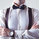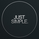1355 просмотров
BO - RSI - M5 Backtesting -Rule of Strategy

A. Data
1. Chart M5 IDC
2. Symbol: EURJPY

B. Indicator
1. RSI

C. Rule of Signal
1. Put Signal
* Rsi create a temporary peak over Extreme Top
row61: peak_rsi= rsi>rsi and rsi>rsi and rsi<rsi and rsi>rsi_top
2. Call Signal
* Rsi create a temporary bottom under Extreme Bottom
row62: bott_rsi= rsi<rsi and rsi<rsi and rsi>rsi and rsi<rsi_bot

D. Rule of Order